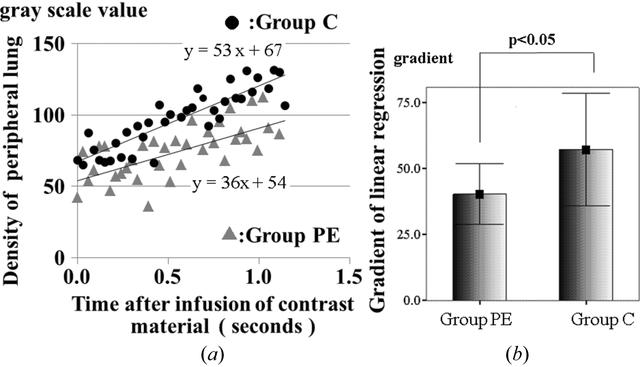disable zoom     view article Figure 3 (a) In the initial 1.2 s, each plot of the average in the two groups can apply to the linear regression line. Each of the regression lines was confirmed as statistically significant (the R2 value of groups PE and C were 0.447 and 0.779, respectively; p < 0.0001). (b). This figure was composed using seven slopes of linear regression lines in both groups. The slope of the linear regression lines in group PE (40.2 ± 11.5) was lower than that in group C (57.1 ± 21.3), a significant difference (p < 0.05). This indicated lower pulmonary blood flow in PE.JOURNAL OFSYNCHROTRONRADIATION
ISSN: 1600-5775
Volume 20| Part 2| February 2013| Pages 376-382